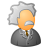# QlikView App Dev

Discussion Board for collaboration related to QlikView App Development.

Announcements
Our next Qlik Insider session will cover new key capabilities. Join us August 11th REGISTER TODAY
cancel
Showing results for
Did you mean:Creator

## Set analysis to variables

Hi all,

I need to use a variable and  filter it with set analysis (in a pivot table) and I cant come to how to do it.

The variable is the calculation of a metric(Performance), all the fields of a particular dimension (Product Line) have one vale of the metric associeted. What i need is to calculate the average performance for 3 specific product lines (A , B , C), the 3 product lines together must be represented with 1 single name 'Total GSS' which its performance= avg(performance A , B, ,C ).

The variable is the following:

vCSAT=sum(TCE_RSLT_METRIC_CSAT_FLAG*if(vWithWeights=1,TCE_RSLT_WEIGHTS,1))/sum(TCE_RSLT_METRIC_CSAT_DSAT_TOTAL_FLAG*if(vWithWeights=1,TCE_RSLT_WEIGHTS,1));

As a dimension I am using:

'GSS'

expression:

// This is just a tryal, that is why i am only tring to filter by 1 Product Line. Nevertheless the objective is the descrived above.

avg({\$<Product Line='A'>} \$(vCSAT))

Thanks a lot for you time and help.

If something is not clear just ask it and i will answer asap.

Regards,

Lluís ^^

1 Solution

Accepted SolutionsPartner

It's hard to provide an answer on a random number.

Have a look at this example and let me know if I am anywhere near where your answer should be.

Really without a target I'm working in the dark.

Good luck

Oscar

25 RepliesMaster II

avg({\$<Product Line={'A'},TCE_RSLT_WEIGHTS={"=\$(vCSAT)"}>} \$(vCSAT))

Not sure if the above expression would work

You are getting products with A from \$(vCSAT)???Partner

You cannot have nested aggregations without using the AGGR function.

From help:

aggr ([ distinct | nodistinct ] [{set_expression}]expression {, dimension})

Returns a set of values of expression calculated over dimensions. The result can be compared to the expression column of a 'local chart', evaluated in the context where the aggr function resides. Each dimension must be a single field. It cannot be an expression (calculated dimension).

If the expression argument is preceded by the nodistinct qualifier, each combination of dimension values may generate more than one return value, depending on underlying data structure. If the expression argument is preceded by the distinct qualifier or if no qualifier is used at all, each combination of dimension values will generate only one return value.

By default, the aggregation function will aggregate over the set of possible records defined by the selection. An alternative set of records can be defined by a Set Analysis expression.

By using this function in Add calculated dimension... it is possible to achieve nested chart aggregation in multiple levels. See also Nested Aggregations and Related Issues.

When used in chart expressions it is possible to achieve Sum of Rows in Pivot Tables.

Examples:

aggr( sum(Sales), Country )

aggr( nodistinct sum(Sales), Country )

aggr( sum(Sales), Country, Region )

count( aggr( sum(Sales), Country ))

Good luck

OscarCreator
Author

First of all thanks for your time.

Sadly it is still not working :S.

Yes, vCSAT have values in A if that is what you mean.Partner

Can you share some sample data with expected results?

Maybe then we can put something together for you.Creator
Author

Sadly I can not share the data I have as it is from a client of the company where i work. And it comes from a database where i do not have direct access.

Sorry and thanks! If you need it i will explain any doubt about the problem.Partner

But you can mock up some fake data to behave similar to the problem you are having.  You would never want to put real data on the community.

Then based upon that data explain what the results should be and we can try to help you with your problem.  Right now all I can do is guess.

Thanks

OscarPartner

So the problem you are having is with your variable.  The variable acts as a replacement value.  If you copy the contents of your variable and paste it into your expression the expression will fail.  The reason it fails is because you cannot do a nested aggregation in an expression without using the AGGR.  In order to use the AGGR you need to also define at what dimension(s) you want to choose.

So you may try something like this:

avg({\$<Product Line='A'>}  Aggr( \$(vCSAT), GSS ))

But again this is simply a guess at this point.

Good luck

OscarMaster II

First create a copy of your original

Then load only top 1000 records from each table

Scramble the data in front end thru Document Properties>Scrambling>>>Creator
Author

I have made an excel with sample data. I give you the excel and the qlik with the table that i can't get working.

The result that i want is:

Showing only the sum of the metric vCSAT for the three product lines (A,B,C). This sum is called GSS.

The TCE_RSLT_METRIC_CSAT_DSAT_TOTAL is only counting how many lines have a value for TCE_RSLT_METRIC_CSAT.

Remember the variable: (it is also in the qlik)

SET vCSAT= sum(TCE_RSLT_METRIC_CSAT_FLAG*if(vWithWeights=1,TCE_RSLT_WEIGHTS,1))/sum(TCE_RSLT_METRIC_CSAT_DSAT_TOTAL_FLAG*if(vWithWeights=1,TCE_RSLT_WEIGHTS,1));